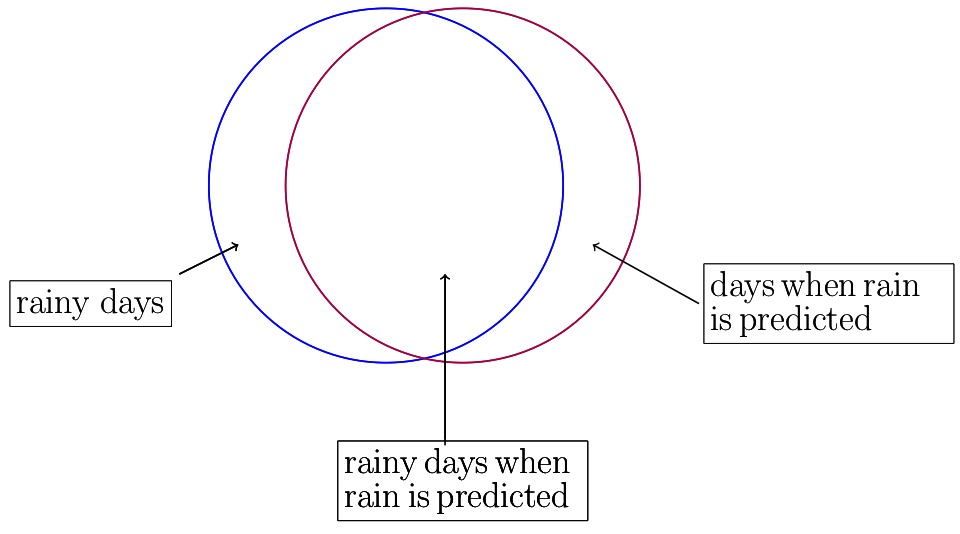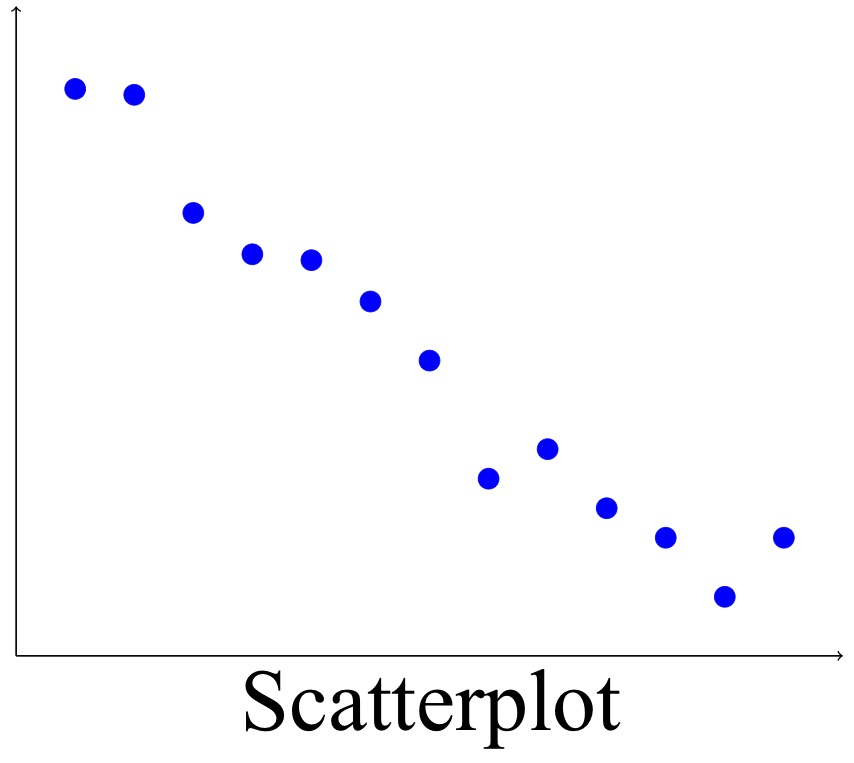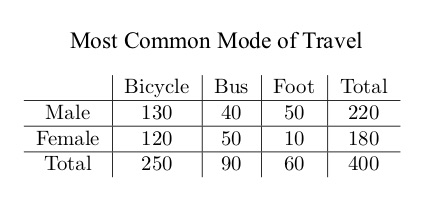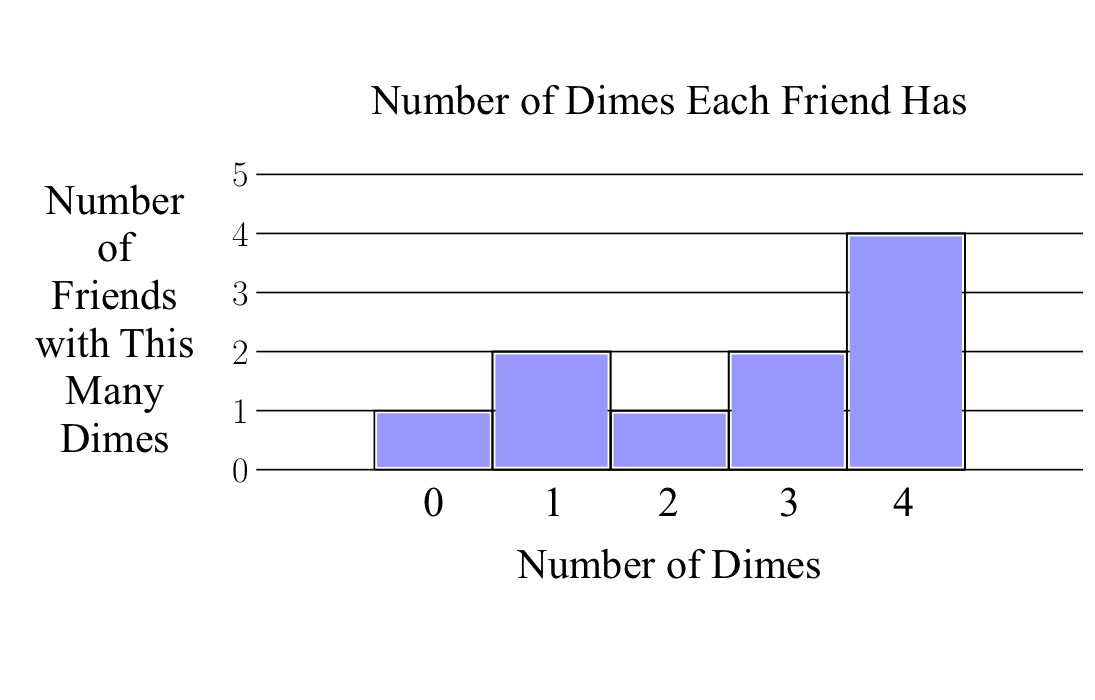# Just Enough Stats for the SAT

You want to go to college but maybe math is not your thing and maybe you’ve never had a chance to learn much of statistics. But still you need to make a decent showing on the SAT. You don’t have time to go down the rabbit hole of a full stats course but you do need some statistics for SAT prep. If that’s you, you’ve come to the right page.

I’ve reviewed the stats questions in the math sections of The Official SAT Study Guide, 2016 edition, to see just what is likely to come up. That is covered on this page.

## Concepts

### Conditional ProbabilityThe probability that one thing will happen when it is known that another thing has already happened. (See probability below.) For example, perhaps the probability that there will be rain on any given day is 1 in 2, or 0.5. On the other hand, perhaps there is rain on 90% of the days on which it is predicted. That means the probability of rain given that rain is predicted is 90%.

### Confidence IntervalSee margin of error below. A confidence interval is a range of likely values for the thing you are trying to estimate. In the example given below for margin of error, the confidence interval is 52% to 58%. That means the number of votes for the candidate is 95% (or some other number determined by statistical methods) likely to be between 52% and 58%.

### Correlation

A relationship between two variables in which one goes up as the other goes up (positive correlation) or one goes up as the other goes down (negative correlation).

### HistogramA graph using bars to show frequency.

### Independent Probabilities

When the probability of two things A and B happening together equals the product of the probabilities of each of those things happening alone — that is, , the probabilities of those two things are said to be independent.

### Line of best fit

The straight line that is closest to all the points on a scatterplot.Think of a dart board that looks like the diagram with the circles. Any dart you throw is as likely to land on any place on the board as on any other. If the “rain” circle overlaps 90% of the “rain predicted” circle, then a dart that lands inside the “rain predicted” circle is 90% likely to land inside the “rain” circle. That is conditional probability.

The first sample problem below is about conditional probability, even if it the problem doesn’t use that term. The percent of respondents that are females who bicycles is the probability that a respondent is female given that the respondent bicycles. The percent of female respondents that are bicyclists is the probability that a respondents a bicyclist given that she is female.

It would be nice if a confidence interval could tell you the limits of what’s possible. But it can’t. It can tell you only the limits of what’s likely.

### Margin of error

A measure of the limits to how far off a statistical estimate is likely to be. The statisticalestimate is the best guess for the actual value. But that number is just an estimate, and numbers close it could be the actual value. The farther a number is from the statistical estimate, the less likely it is to be the actual value. The margin of error is a reasonable limit to how far the margin of error is likely to be from the statistical estimate.

### Mean

In common parlance, this is the average. It is the sum of all numbers in a set divided by the number of numbers in that set. For example, the mean of the set {1, 2, 3} is .

### Median

This is the number that comes out in the middle when the numbers in a set are lined up in order. If the number of numbers in the set is even, the median is the average of the two middle numbers. The median of the set {1, 2, 3} is 2 and the median of the set {1, 2, 3, 4} is 2 1/2.

### Outlier

A point in a data set that is so far away from other data points that it does not seem consistent. For example, if you are shopping for cat food and there are thirty different kinds, with an average price per can of about 75 cents, and only one price is over $1.50, then if that high price is$75, it is an outlier. Maybe someone forgot a decimal point.

### Probability

An outcome’s frequency over the long term. For example, if you flip a fair coin 1000 times, something close to half your flips will be heads and the other half will be tails, and the probability of either a head or a tail is 1/2.

You’ve most likely seen the concept margin of error in polls before an election. If a poll says candidate A is projected to get 55% of the vote with a margin of error of +/- 3 points with 95% confidence, that means candidate A is about 95% certain to get somewhere between 52% and 58% of the vote. The margin of error is the farthest that the actual number is likely to be from the statistical estimate — in this case, 3 points.

What statisticians call the mean is what everyone else calls the average.

### Scatterplot

A graph based on the coordinate plane, with one variable represented on the x-axis and the other on the y-axis. A dot represents each data point.

### Standard deviation

This is a measure of how spread out the data in a set are. For comparison, think of a simpler measure of spread: range, the difference between the greatest and the least numbers in a set. For example, the range of the set {1, 2, 3, 4} is  Range considers only the most extreme values. Standard deviation, on the other hand, considers all values but gives more weight to numbers far from the mean than to numbers close to the mean. There is a formula for standard deviation, but I don’t think you need to know it — I did not see any practice SAT questions that require you to calculate a standard deviation.Got a stats-related problem that isn’t covered here? .

For one more prob-stats problem, see the last sample problem on the Word Problems page.

## Sample Problems

### Sample Problem: Reading a Mean from a Table

Question. A survey of 400 people was done to find the most common mode of transportation for students who don’t drive (and are not driven). The results are shown in the table. What percent of respondents are females who bicycle? What percent of female respondents are bicyclists? If rounding is necessary, round your answer to the nearest percent.Solution.

• Percent of respondents that are females who bicycle? That’s the number of females who bicycle divided by all the respondents, expressed as a percent. The number of females who bicycle is the number in the cell in the “bicycle” column and in the “female” row: 120. The total number of respondents is in the bottom right cell of the table: 400. 120/400  = 30%.
• Percent of female respondents who are bicyclists: That’s the number of female bicyclists — 120 — divided by the number of female respondents — 180 — expressed as a percent. That’s 120/180 = 67%.

### Sample Problem: Reading a Mean from a Histogram

Question. A group of ten friends got together and played a card game, using dimes for their bets.The number dimes that each person had at the end of the evening is shown in the histogram. To the nearest whole number, what is the mean number of dimes that the friends ended with?

Solution. The mean is the sum of the numbers divided by the number of numbers. There are:

• one person with no dimes,
• two people with 1 dime each,
• one person with 2 dimes,
• two people with 3 dimes each, and
• four people with 4 dimes each

for a total of ten friends. Then the mean is

To the nearest whole number, that’s 3. On average they ended up with three dimes each. Roughly.

(This problem is like problem #12  on p. 378 of The Official SAT Study Guide.)

### Sample Problem: Reading from a ScatterplotQuestion. A store offers various styles of dresses at a variety of prices. The store manager made a scatterplot, shown, of the price of each dress style v. the number sold during one week, and found a negative correlation. The scatterplot is shown to the left. The line of best fit is also shown. For the dress priced at $150, approximately what is the difference between the actual number sold and the number indicated by the line of best fit? Solution. It’s hard to get an exact reading from a graph, but in this case an approximate reading is good enough. It looks like about twenty dresses were sold for$150. For a price of \$150, the line of best fit seems to show about 2. Then the difference between the actual price and the line of best fit is about 20 – 2 = 18.

### Sample Problem: Mean, Median, Outlier

Question. The dotplot below shows exam scores in a statistics class with 23 students. Each blue dot represents one student’s score. For example, two students scored 75 and three scored 80. The score of 40 is an outlier, meaning it’s quite far away from the rest of the data. (During the exam, not only did that student’s pen run out, but also his calculator died and he ran out of leads for his mechanical pencil.) The teacher will give that student a retake and will discard that grade. The teacher has already calculated the mean, median, and range of the scores. Which of these values will change the most when the score of 40 is removed?Solution. Let’s take these things — mean, median, and range — one at a time.

#### Mean

The mean is the sum of the numbers divided by the number of numbers. The mean of the numbers shown in the dotplot is 85.65. Take out the 40 grade and the mean goes up to 87.73. Take out the 40 and the mean should go up by 2.08 points.

#### Median

This is the number that comes out in the middle when the numbers are lined up in order. The dotplot lines them up for you. There are 23 numbers, so the median is twelfth. Starting from either end of the dotplot, find the twelfth dot. It’s at 90. For the original set the median is 90.

What happens when the 40 comes out? Now there are 22 numbers, so the median is the average of the middle two: the eleventh and the twelfth. From the dotplot it looks like the eleventh and twelfth numbers are both 90, so the new median is also 90. Taking out the 40 has not changed the median at all.

#### Range

This is the difference between the highest and the lowest values. For the original set of numbers, the range is 100 – 40 = 60. For the set of numbers with 40 removed, the range is 100 – 75 = 25. That’s a change of 60 – 25 = 35. Taking out the 40 has decreased the range by 35 points.

Answer: Taking the outlier out changes the range more than it changes either the mean or the median.

The math of this question is based on question #14 on p. 379 of The Official SAT Study Guide.

For this problem, the mean is cumbersome to calculate from the dotplot. If you’re only trying to compare the change in the mean, the change in the range, and the change in the median, and if the values are not too close together, then an estimate, even a rough one, may do.

Look at the dotplot and consider the set of numbers without the 40 included. The graph is almost symmetric around a vertical line between 85 and 90. If it were perfectly symmetric, the mean would be halfway between 85 and 90: 85.5. But there are more dots above 90 than above 85, so the mean is higher. You can estimate, roughly, that the mean of the numbers without 40 is 90.

What if 40 were there? How much could it change the mean? It will pull the mean down by (roughly) its difference from the mean divided by the number of numbers in the set. (This estimate is quite approximate, but in a way that’s the point.) That’s (90 – 40)/23, or 50/23, which is a bit more than 2. So you can expect the removal of the 40 to bring the mean up by about 2 points, maybe a little more. That is consistent with the more exact number, 2.08. Since 2 points or even 3 or 5 points, is far less than the 35 points by which the removal of the 40 changes the range, this estimation is good enough. It saves a lot of time from calculating the mean exactly.

### Sample Problem: Standard Deviation

Question. Two discount stores sell socks in packs of twelve pairs. The prices and the number of pairs of socks at each price are shown below. Which set of prices — those in store A or those in store B — has the greater standard deviation?

 Price Kinds of Packages at That Price in Store A Kinds of Packages at That Price in Store B 13 2 5 14 5 4 15 6 2 16 5 4 17 2 5

Solution. Remember that standard deviation measures the extent to which data are spread out. That is determined by two things: the range (the difference between the greatest number and the least) and the way the numbers are distributed within the range. More data near the extremes of the range means a higher standard deviation. For this problem, the range is the same for both stores, so any difference in standard deviation will come from the distribution of those numbers within the set. Note that in store A the greatest numbers of packages are near the center of the range and at store B the greatest numbers are at the ends of the range. So the data set for store B has the higher standard deviation. (p. 721, #23)